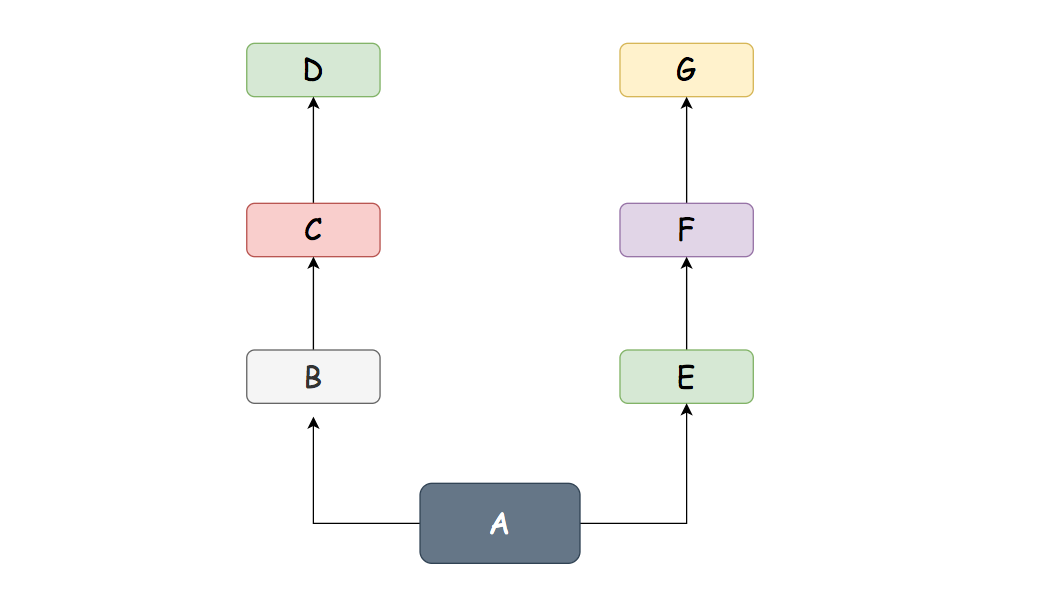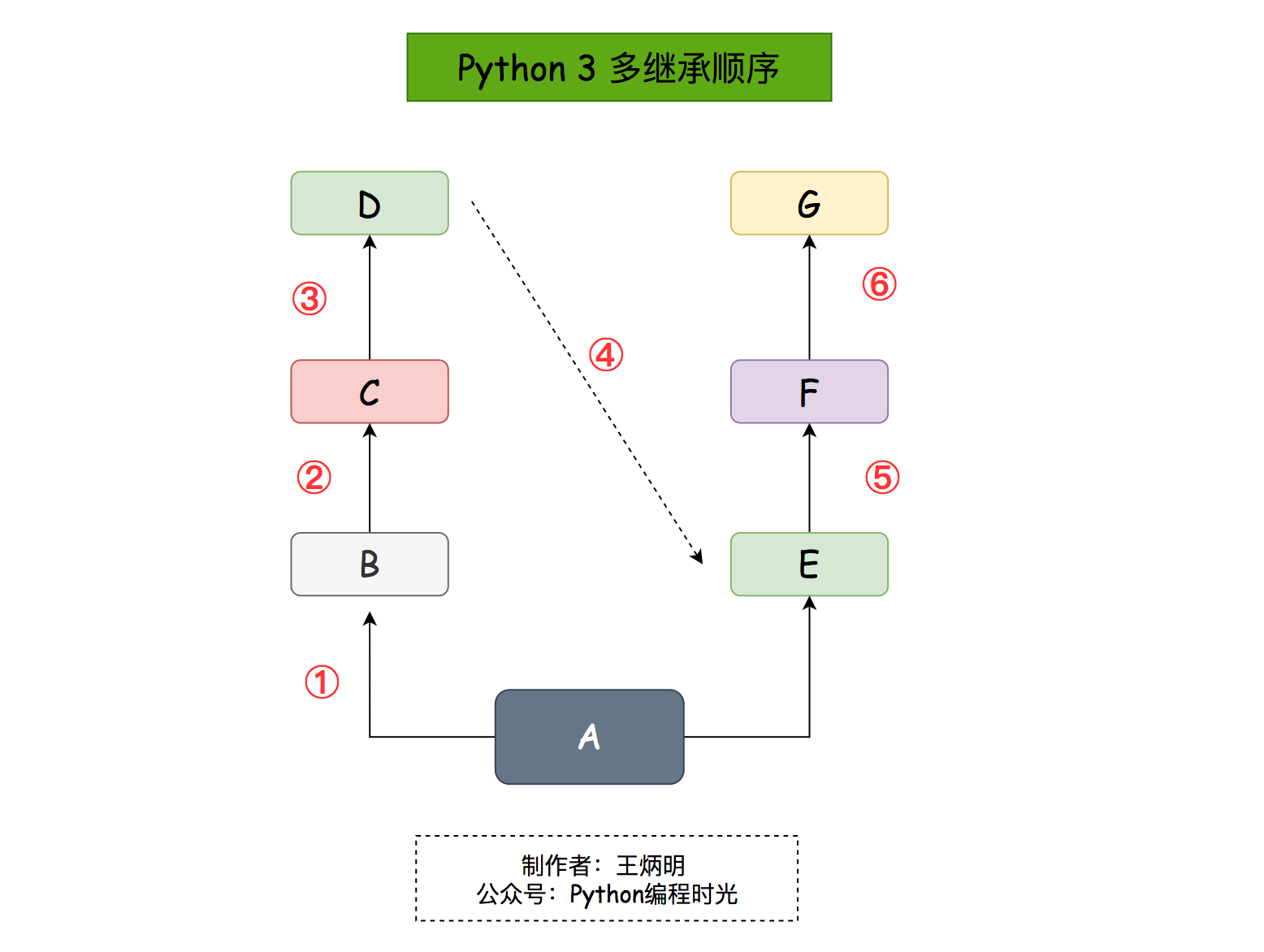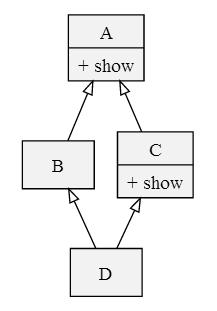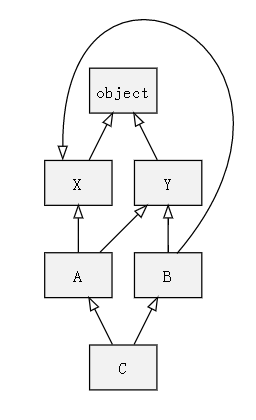# 零基础学 Python（40）：类的继承（Inheritance）

class 子类(父类):


## 1. 单继承

# 父类定义
class People:
def __init__(self, name, age, weight):
self.name = name
self.age = age

def speak(self):
print(f"{self.name} 说: 我{self.age}岁。")

# 单继承示例
class Student(People):
def __init__(self, name, age, weight, grade):
# 调用父类的实例化方法
People.__init__(self, name, age, weight)


>>> xm = Student(name="小明", age=10, weight=50, grade="三年级")
>>> xm.speak()



# 单继承示例
class Student(People):
def __init__(self, name, age, weight, grade):
# 调用父类的实例化方法
People.__init__(self, name, age, weight)

# 重写父类的speak方法
def speak(self):


>>> xm = Student(name="小明", age=10, weight=50, grade="三年级")
>>> xm.speak()



## 2. 多继承

Python 还支持多继承，可以继承自多个类。

class 子类(父类1, 父类2, 父类3...):


class D:pass
class C(D):pass

class B(C):
def show(self):
print("i am B")

class G:pass
class F(G):pass

class E(F):
def show(self):
print("i am E")

class A(B, E):pass>>> a = A()
>>> a.show()
i am B


## 3. 继承顺序

class D:
def show(self):
print("i am D")

class C(D):pass
class B(C):pass

class G:pass
class F(G):pass

class E(F):
def show(self):
print("i am E")

class A(B, E):pass


>>> a = A()
>>> a.show()
i am D## 4. MRO 算法

• 从左向右
• 深度优先

1. 使用 __mro__ 来查询
2. 使用 merge算法进行推导

### 使用 mro 查询

class A(object):pass
class B(A):pass
class C(A):pass
class D(B, C):pass>>> print(D.__mro__)


>>> import inspect
>>> print inspect.getmro(D)


(<class '__main__.D'>,
<class '__main__.B'>,
<class '__main__.C'>,
<class '__main__.A'>,
<class 'object'>)


### 使用 merge 推导1. 检查第一个列表的头元素（如 L[B1] 的头），记作 H。
2. 若 H 未出现在其它列表的尾部，则将其输出，并将其从所有列表中删除，然后回到步骤1；否则，取出下一个列表的头部记作 H，继续该步骤。
3. 重复上述步骤，直至列表为空或者不能再找出可以输出的元素。如果是前一种情况，则算法结束；如果是后一种情况，说明无法构建继承关系，Python 会抛出异常。

L[object] = [object]
L[D] = [D, object]
L[E] = [E, object]
L[F] = [F, object]
L[B] = [B, D, E, object]
L[C] = [C, D, F, object]
L[A] = [A] + merge(L[B], L[C], [B], [C])
= [A] + merge([B, D, E, object], [C, D, F, object], [B], [C])
= [A, B] + merge([D, E, object], [C, D, F, object], [C])
= [A, B, C] + merge([D, E, object], [D, F, object])
= [A, B, C, D] + merge([E, object], [F, object])
= [A, B, C, D, E] + merge([object], [F, object])
= [A, B, C, D, E, F] + merge([object], [object])
= [A, B, C, D, E, F, object]


## 附录：参考文章